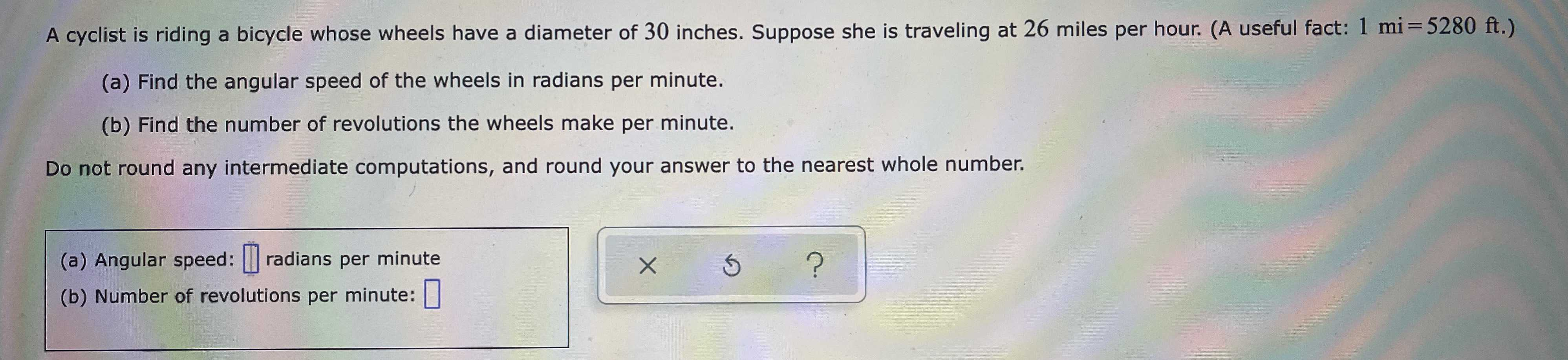### ¿Todavía tienes preguntas de matemáticas?

Pregunte a nuestros tutores expertos
Algebra
PreguntaA cyclist is riding a bicycle whose wheels have a diameter of $$30$$ inches. Suppose she is traveling at $$26$$ miles per hour. (A useful fact: $$1$$ mi $$= 5280 ft$$ .)

(a) Find the angular speed of the wheels in radians per minute.

(b) Find the number of revolutions the wheels make per minute.

Do round any intermediate computations, and round your answer to the nearest whole number.

(a) Angular speed: (b) Number of revolutions per minute: